TitleCollege Algebra
Tutorial 6: PolynomialsLearning Objectives

 After completing this tutorial, you should be able to: Identify a term, coefficient, constant term, and polynomial. Tell the difference between a monomial, binomial, and trinomial. Find the degree of a term and polynomial. Combine like terms. Add and subtract polynomials. Multiply any polynomial times any other polynomial. Use the FOIL method to multiply a binomial times a binomial. Use special product rules to multiply a binomial squared and a product of a sum and difference of two terms.Introduction

 In this tutorial we will be looking at the different components of polynomials.  Then we will move on to adding, subtracting and multiplying them.  Some of these concepts are based on ideas that were covered in earlier tutorials.  A lot of times in math you use previous knowledge to learn new concepts.  The trick is to not reinvent the wheel each time but recognize what you have done before and draw on that knowledge to help you work through the problems.Tutorial

 Let’s start with defining some words before we get to our polynomial.

 Term

 A term is a number, variable or the product of a number and variable(s).  Examples of terms are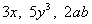, z.

 Coefficient

A coefficient is the numeric factor of your term.

Here are the coefficients of the terms listed above:

 Term Coefficient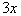3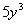5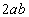2 z 1

 Constant Term

 A constant term is a term that contains only a number. In other words, there is no variable in a constant term.  Examples of constant terms are 4, 100, and -5.

 Standard Form of a Polynomial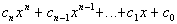where n is a non-negative integer.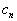is called the leading coefficient.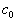is a constant.

 In other words, a polynomial is a finite sum of terms where the exponents on the variables are non-negative integers.  Note that the terms are separated by +'s and -'s. An example of a polynomial expression is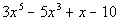.

 Degree of a Term

 The degree of a term is the sum of the exponents on the variables contained in the term.  For example, the degree of the termwould be 1 + 1 = 2.  The exponent on a is 1 and on b is 1 and the sum of the exponents is 2. The degree of the termwould be 3 since the only variable exponent that we have is 3.

 Degree of the Polynomial

 The degree of the polynomial is the largest degree of all its terms.

 Descending Order

 Note that the standard form of a polynomial that is shown above is written in descending order.  This means that the term that has the highest degree is written first, the term with the next highest degree is written next, and so forth.  Also note that a polynomial can be “missing” terms.  For example, the polynomial written above starts with a degree of 5, but notice there is not a term that has an exponent of 4.  That means the coefficient on it is 0, so we do not write it.

 Some Types of Polynomials

 Type Definition Example Monomial A polynomial with one term 5x Binomial A polynomial with two terms 5x - 10 Trinomial A polynomial with three terms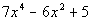Example 1:   Find the degree of the polynomial and indicate whether the polynomial is a monomial, binomial, trinomial, or none of these: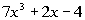.

 Since the degree of the polynomial is the highest degree of all the terms, it looks like the degree is 3.  Since there are three terms, this is a trinomial.Example 2:   Find the degree of the polynomial and indicate whether the polynomial is a monomial, binomial, trinomial, or none of these: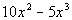.

 Since the degree of the polynomial is the highest degree of all the terms, it looks like the degree is 3.  Make sure that you don’t fall into the trap of thinking it is always the degree of the first term.  This polynomial is not written in standard form (descending order).  So we had to actually go to the second term to get the highest degree.   Since there are two terms, this is a binomial.Example 3:   Find the degree of the polynomial and indicate whether the polynomial is a monomial, binomial, trinomial, or none of these:   -20.

 Since the degree of the polynomial is the highest degree of all the terms, it looks like the degree is 0.  Since there is one term, this is a monomial.

 Combining Like Terms

 Recall that like terms are terms that have the exact same variables raised to the exact same exponents.  One example of like terms is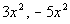.  Another example is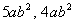. You can only combine terms that are like terms.  You can think of it as the reverse of the distributive property. It is like counting apples and oranges.  You just count up how many variables  you have the same and write the number in front of the common variable part.

 Step 1:   Remove the ( ) .

 If there is only a + sign in front of ( ), then the terms inside of ( ) remain the same when you remove the ( ). If there is a - in front of the ( ), then distribute it by multiplying every term in the ( ) by a -1 (or you can think of it as negating every term in the ( )).

 Step 2:  Combine like terms.Example 4:   Perform the indicated operation and simplify: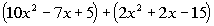.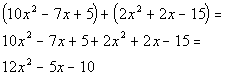*Remove the (  ) *Add like terms togetherExample 5:   Perform the indicated operation and simplify: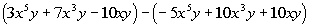.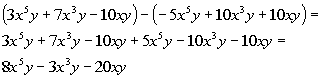*Dist. the - through second ( ) *Combine like terms

 Multiplying Polynomials

 In general, when multiplying two polynomials together, use the distributive property until every term of one polynomial is multiplied times every term of the other polynomial.  Make sure that you simplify your answer by combining any like terms. On this page we will look at some of the more common types of polynomials to illustrate this idea.

 (Monomial)(Monomial)

 In this case, there is only one term in each polynomial.  You simply multiply the two terms together.Example 6:   Find the product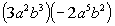.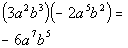*Mult. like bases add exp.

 (Monomial)(Polynomial)

 In this case, there is only one term in one polynomial and more than one term in the other.  You need to distribute the monomial to EVERY term of the other polynomial.Example 7:   Find the product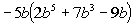.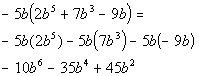*Dist. -5b *Mult. like bases add exp.

 (Binomial)(Binomial)

In this case, both polynomials have two terms.  You need to distribute both terms of one polynomial times both terms of the other polynomial.

One way to keep track of your distributive property is to use the FOIL method.   Note that this method only works on (Binomial)(Binomial).

 F First terms O Outside terms I Inside terms L Last terms

This is a fancy way of saying to take every term of the first binomial times every term of the second binomial.  In other words, do the distributive  property for every term in the first binomial.Example 8:   Find the product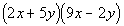.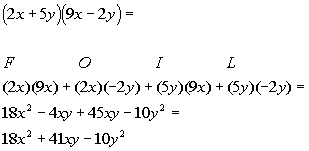*Use the FOIL method *Combine like terms

 Binomial Squared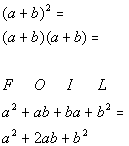Special product rule for  a binomial squared: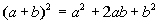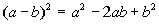In other words, when you have a binomial squared, you end up with the first term squared plus (or minus) twice the product of the two terms plus the last term squared. Any time you have a binomial squared you can use this shortcut method to find your product. This is a special products rule.  It would be perfectly ok to use the foil method on this to find the product.  The reason we are showing you this form is that when you get to factoring, you will have to reverse your steps.  So when you see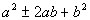, you will already be familiar with the product it came from.Example 9:   Find the product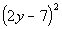.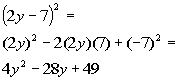*Product of the sum and difference  of two terms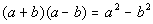This is another special products rule.  It would be perfectly ok to use the foil method on this to find the product.  The reason we are showing you this form is that when you get to factoring, you will have to reverse your steps.  So when you see a difference of two squares, you will already be familiar with the product it came from.Example 10:   Find the product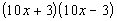.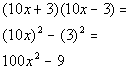*(Polynomial)(Polynomial)

 As mentioned above, use the distributive property until every term of one polynomial is multiplied by every term of the other polynomial.  Make sure that you simplify your answer by combining any like terms.Example 11:   Find the product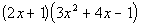.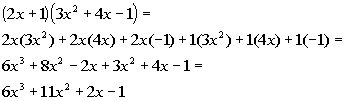*Use Dist. Prop. twice *Combine like terms

 Special product rule for  binomial cubed: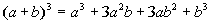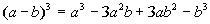In other words, when you have a binomial cubed, you end up with the first term cubed plus (or minus) three times the first term squared times the second term plus three times the first term times the second term squared plus (or minus) the last term cubed. Any time you have a binomial cubed you can use this shortcut method to find your product.Example 12:   Find the product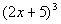.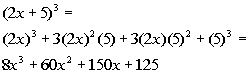*Practice Problems

 These are practice problems to help bring you to the next level.  It will allow you to check and see if you have an understanding of these types of problems. Math works just like anything else, if you want to get good at it, then you need to practice it.  Even the best athletes and musicians had help along the way and lots of practice, practice, practice, to get good at their sport or instrument.  In fact there is no such thing as too much practice. To get the most out of these, you should work the problem out on your own and then check your answer by clicking on the link for the answer/discussion for that  problem.  At the link you will find the answer as well as any steps that went into finding that answer.Practice Problems 1a - 1c: Find the degree of the polynomial and indicate whether the polynomial is a monomial, binomial, trinomial, or none of these.

 1b.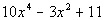(answer/discussion to 1b) 1c.(answer/discussion to 1c)Practice Problems 2a - 2e: Perform the indicated operation.

 2a.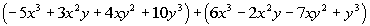(answer/discussion to 2a) 2b.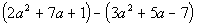(answer/discussion to 2b)

 2c.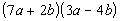(answer/discussion to 2c) 2d.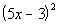(answer/discussion to 2d)

 2e.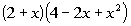(answer/discussion to 2e)Need Extra Help on these Topics?

The following are webpages that can assist you in the topics that were covered on this page:

 http://www.wtamu.edu/academic/anns/mps/math/mathlab/int_algebra/int_alg_tut25_poly.htm This webpage goes over the basic terminology of polynomials as well as how to add and subtract them. http://www.purplemath.com/modules/polydefs.htm This webpage helps you with the different parts of a polynomial. http://www.purplemath.com/modules/polyadd.htm This webpage helps you with adding and subtracting polynomials. http://www.wtamu.edu/academic/anns/mps/math/mathlab/int_algebra/int_alg_tut26_multpoly.htm This webpage goes over multiplying polynomials. http://www.algebrahelp.com/lessons/simplifying/distribution/ This website helps with the distributive property. http://www.algebrahelp.com/lessons/simplifying/foilmethod/ This website helps with the FOIL method and (polynomial)(polynomial). http://www.purplemath.com/modules/polymult.htm This webpage helps with multiplying polynomials.

Go to Get Help Outside the Classroom found in Tutorial 1: How to Succeed in a Math Class for some more suggestions.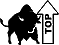Videos at this site were created and produced by Kim Seward and Virginia Williams Trice.
Last revised on Dec. 13, 2009 by Kim Seward.